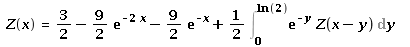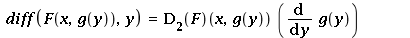6 years, 6 days

## collocation method for integral equation...

Maple 2015

Hello,

I am looking help for solving this integral equation using the collocation method with 1.5<= x<=3.5 ?I have used the successive approximation method and the solution seems to be increasing.

Thanks

## derivative of a function depending on a ...

Maple 2015

Hello,

I have the general function F(x,g(y)), and I don't understand the following notation of the derivative of  F w.r.t  y in Maple ?  What is D2(F) ?  Can we provide a concrete example ?Thank you!

## Showing numbers in y-axis...

Maple 2015

Hello,

How to show the y-axis numbers in the second plot ?   y is in [0,3].

I try the following but it shows a negative axis.

display(P, L, axis = [tickmarks = [5, subticks = 3], thickness = 2]);  this is not correct

The file is attached.

Thanks

## How to iterate an Integral equation depe...

Maple 2015

Hello,

I need help to approximate Fn(x,y) and Sn(y) for n>=3 to see the convergence.

Here is the problem:

I have an integral equation Fn(x, y) defined by

F0(x,y) = 0,

F1(x,y) = (9/8)*(y+1) - (21/8)*( y*exp(-2*x) + exp(-x) )

Fn+1(x,y) = (9/8)*(y+1) - (21/8)*( y*exp(-2*x) + exp(-x) ) + (3/4)*int ( Fn( x-z, 2*y*exp(-z) ), z=0..Un(x) ), where the function Un(x) is defined below.

where I suppose that Fn(x,y) >=0 and y>=0 and x>=0. I am iterating the integral equation and find the zeros Sn(y) solutions of Fn(S(y) , y) = 0. The goal is to show that the sequence Fn(x,y) converges to some F(x,y) and Sn(y) converges to some S(y). We can also plot the curves Sn(y) to show the convergence. Notice that Sn(y) >= 0 is increasing in n and decreasing in y.

What I did :

If n = 0, I solve F1(x,y) = 0 and find analytically x = S1(y) = -ln((1/14)*(-7+sqrt(84*y^2+84*y+49))/y). Notice that F1(x,y) = 0 is quadratic in exp(-x) and then F1(x,y) = 0 is easy to solve. S1(y) is the positive function for y>=0.

If n = 1, we need to solve F2(x,y) = (9/8)*(y+1) -(21/8)*( y*exp(-2*x) + exp(-x) ) + (3/4)*int ( F1( x-z, 2*y*exp(-z) ), z, 0, U1(x) ). The function U1(x) is obtained as follows :

i) We define g( U ) = U + S1( 2*y*exp(-U) )

ii) We find the function U1(x,y) solution of g( U1(x,y) ) = x

A generalization of Un(x) is given by

i) Define g( U ) = U + Sn( 2*y*exp(-U) )

ii) Find the function Un(x,y) solution of g( Un(x,y) ) = x

The curve S2(y) :

I find the analytic function F2(x, y), but it is impossible to solve F2(x,y) = 0 and find x = S2(y) analytically. So I choose an interval [0, 10] for y and discretize that interval. Now at each point yi in [0, 10], the function F2(x, yi) depends only on x. I apply the bisection method (dichotomic method) and find the value xi such that F2(xi, yi) = 0. The data (yi, xi) gives the curve S2(y). I plot the data (yi, xi) and (y, S1(y)) in the same curve.

Question:

It is impossible to iterate the process below and find F3(x, y), F4(x,y), etc... because we cannot find U2(x), U3(x), etc... analytically. Any suggestions or helps to plot the curves (y, Sn(y)) for n = 3, 4, etc. to see the convergence ?

Thanks !

## Newton's method or Bisection method of a...

Maple 15

Hello,

How to write the Newton's method and Bisection method in Maple of a function depending on a parameter ? The goal is to solve the equation F(y, eta) = 0 and find the root y(eta) using the Newton's method or Bisection method. An example is attached.

Thank you1 2 3 4Page 1 of 4
﻿